It is well documented in science history that Michael Faraday was the discoverer of how an electric current will flow in a changing magnetic field. By doing this, an induced ionisation is established in the surface area of the conductor by the force of the magnet dislodging electrons from the outer valence bands of the atoms. This creates a negative current field that will surround the positive conductor and will be attracted to the conductor due to opposites attract. You now have an induced electric field which will in turn induce a magnetic field due to the 'Endothermic Electric Effect.' Not only will the fixed lattice positive ion atoms in the sphere of the conductor attract the negative electron current field, it will also attract electrons from the environment. This is the first action of a separation of charge in an atom which is a direct reaction caused by the force of the magnet  which acts as an EMF input as is the catalyst. The Photoelectric effect to the Endothermic Electric effect are the stages of a growing electric field. ‘The Endothermic Electric Effect’ being the next reaction in electricity after ‘The Photoelectric Effect’. The electron is NOT free to move as it will in the conductor, it is controlled by attraction force and movement is outside of the wire or plate and is directed towards the conductor and load. The reaction becomes exothermic due to friction, this is excess force inwards to the conductor.

The atom{s} ion will vibrate with energy to a certain frequency due to the impact force vibration induced by the magnet’s EMF, and will have a positive charge measured in joules due to an electron being detached from the atom{s} last available energy level.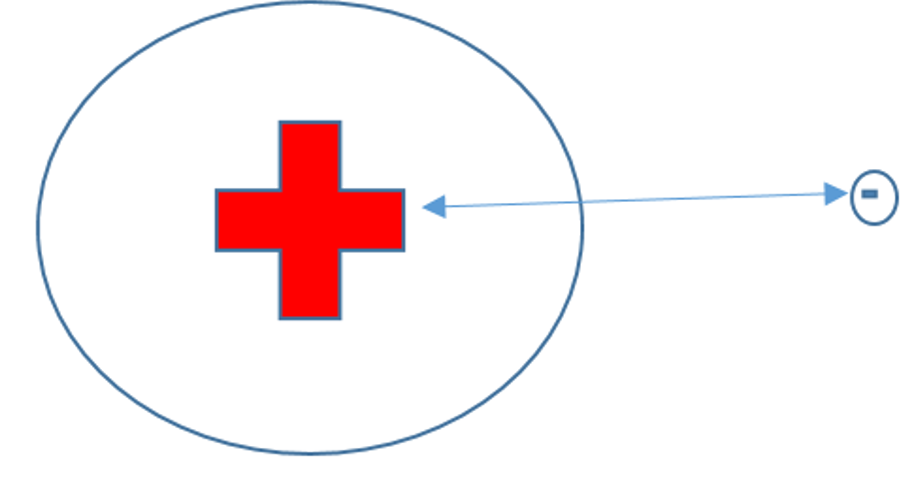Not to scale

Figure58: An atom ion as a diameter around 1850 times bigger than the electron

The atom has altered from its original neutral state and become electrically charged. The initial inward force applied to what becomes an electric field system experiences Newtonian mechanics when confronted with a solid lattice. For example:

If one is to introduce a force at a 90 degrees angle to the conductor, the vibrational force will bounce back in the same direction it came from. Depending on how malleable the surface is and how big the inward force is, will govern the depth of vibrational penetration into the material. In other words, the reaction will depend on the density of the metal lattice and the force applied, these are the first two parameters encountered in the Photoelectric Effect reaction. The newton force is usually described in volts/meters or can be newton /meters or joules, -with respect to time. This reaction ionises atoms due to the impact of two objects, starting a vibration through the system, and an electron bond is broken from the atom and removed to what we term as the negative part of the growing electric field. This is how an electric field of positive and negative opposite charge attraction is established and is an example of mass into energy: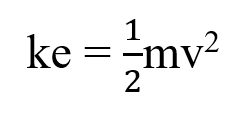“For an electron gun with a voltage between its cathode and anode of V = 100V the electron will have a speed of about v = 6 × 106 m/s. (Relativistic effects have not been taken into account.) There will be no more acceleration once the electrons have passed through the anode. “  The speed of electrons | IOPSpark     

The atom as given the electron to the growing electric field. An electron has a mass of 10-30 kilogram .

The hv or photon in the Photoelectric effect equation, is the electricity equivalent terminology to ‘Electromagnetic Force’ (EMF) placed into the system, this is the catalyst to the energy conversion, and as we know, energy is conserved with both the atom and the electron now having the transferred energy. Atoms in a metal structure need a certain amount of ‘energy’ to dislodge the outer electrons from the metal atoms in the lattice and induce an altered state. It can be seen that the initial pressure or force into the metal will cause a certain amount of electrons to be removed from the atoms of the metal lattice and a positive and negative charge is achieved in the system and the 2 operators required for an electric field to be a force to do work is established.

The rotating magnet in an energy generator will continually exert a force on the negative current field as it rotates or moves, this is also known as the Hall effect [ ] . When measured with a measuring device such as an oscilloscope, you will observe what is known as a ‘sinusoidal wave.’ This is due to the negative current being repulsed by the magnet and attracted to the conductor, then flowing from high to low pressure around the coil which will have a lower pressure per unit area at 90 degrees to the striking point of the magnet.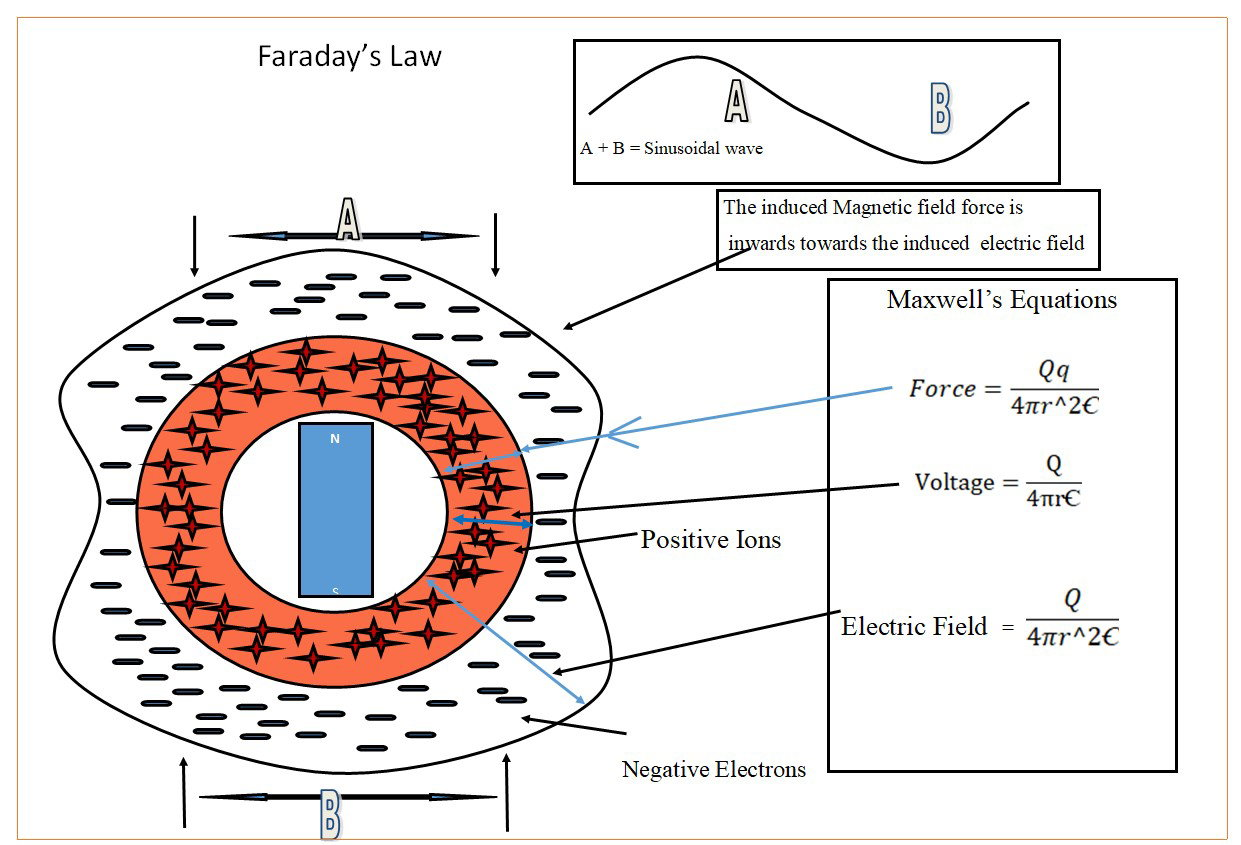The conductor acts as the initial load in an electricity generator.

How does a current flow in a changing magnetic Field?

A changing magnetic field induces a current to flow in a conductor because:

The magnet induces the Photoelectric Effect in the coil.

The force of the magnet and the attractive force between the induced positive and negative of the electric field, create a push and pull effect on the negative electron field of the electric field of the coil.

Why does there have to be a closed loop of wire?

The closed loop of wire acts as a load, which allows the higher pressure of the negative current at the striking point of the magnet to move to a lower pressure along the loop.

The current flow is from the striking point of the magnet to 90o from the striking point of the magnet.

The result is as an alternating current around the loop.

Where does the induced current reside?

The induced current is negative and is only one half of an electric field.

It resides outside the positive atom ions of the wire.

How does a current appear around a wire?

Electrons are expelled out of the atoms of the wire.

Electrons from the surrounding gases of the environment are attracted to the positive atom ions of the wire.

A negative electron current field is induced by the EMF input.

A negative electron current field is an inward attractive force towards the positive atom ions of the wire.

The direction of flow is from high to low pressure along the circuit loop.

# Maxwell’s Equations

We understand electromagnetic theory by using James Maxwell’s mathematical equations which unified the theories of Gauss, Faraday and Ampere.

Maxwell said at his inaugural speech to Kings College,

‘You must apply the principal of electricity to the formula written.’

(Equation 13)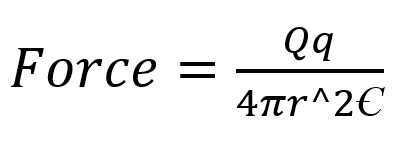F                      = force

Q                     = A positive [+] [Q] voltage attractive force out, of the sphere

q                      = A negative [-] [q] current attractive force in, to the sphere.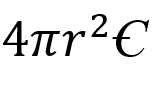Surface area of an electromagnetic field multiplied by the permittivity of free space.

Using this equation in endothermic state of charge it identifies the following:

• There is an induced magnetic field around the induced electric field of the wire created by the EMF of the magnet
• As seen in figure 59 Faraday’s law, the force is inward to the electric field whilst the system is endothermic.
• The Permittivity of free space and a surface area of a sphere of an electromagnetic field.

## Voltage Equation

(Equation 14)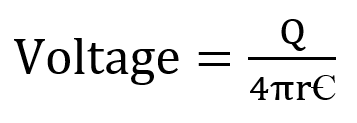This equation expresses and identifies:

1. One of the operators of electricity, Positive atom ion charge that attracts negative charge,
2. The surface area of the conductor sphere
3. Permittivity of free space
4. This equation is only slightly different to the force equation, but the differences are significant.

### Maxwell expresses the electric field

(Equation 15)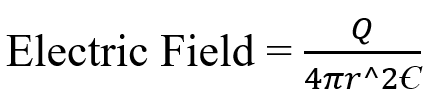You will notice that there is only one charge symbol Q in this equation. Maxwell has expressed an electric field as ‘Q’ only, it is an open voltage equation, with no current flow.

For electricity to exist there is a separation of charge and 2 operators, this represents endothermic electricity, an electric field under load, with direction of force in the magnetic field directed towards the electric field. Also there is a high pressure effect from the magnetic field directed towards the electric field. Refer to Faradays law diagram.